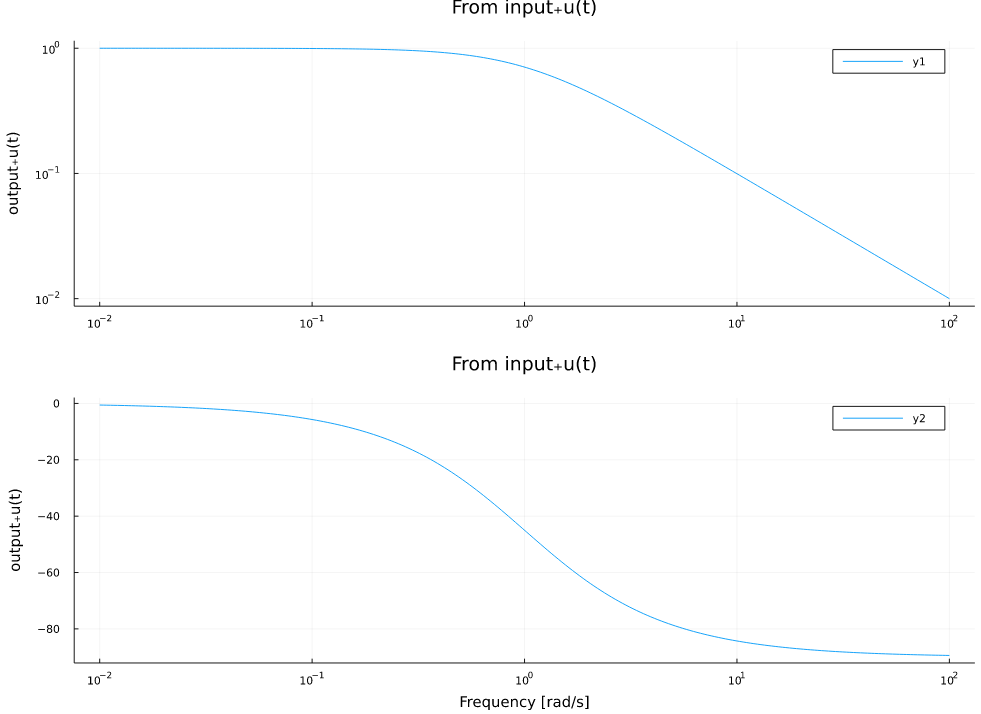# ControlSystemsMTK.jl

ControlSystemsMTK provides an interface between ControlSystems.jl and ModelingToolkit.jl.

See the videos below for examples of using ControlSystems and ModelingToolkit together.

## Installation

pkg> add ControlSystemsMTK

## From ControlSystems to ModelingToolkit

Simply calling ODESystem(sys) converts a StateSpace object from ControlSystems into the corresponding ModelingToolkitStandardLibrary.Blocks.StateSpace. If sys is a named statespace object, the names will be retained in the ODESystem.

### Example:

julia> using ControlSystemsMTK, ControlSystemsBase, ModelingToolkit, RobustAndOptimalControl

julia> P0 = tf(1.0, [1, 1])  |> ss
StateSpace{Continuous, Float64}
A =
-1.0
B =
1.0
C =
1.0
D =
0.0

Continuous-time state-space model

julia> @named P = ODESystem(P0)
Model P with 2 equations
States (3):
x(t) [defaults to 0.0]
input₊u(t) [defaults to 0.0]
output₊u(t) [defaults to 0.0]
Parameters (0):

julia> equations(P)
2-element Vector{Equation}:
Differential(t)(x(t)) ~ input₊u(t) - x(t)
output₊u(t) ~ x(t)

## From ModelingToolkit to ControlSystems

An ODESystem can be converted to a named statespace object from RobustAndOptimalControl.jl by calling named_ss

named_ss(ode_sys, inputs, outputs; op)

this performs a linearization of ode_sys around the operating point op (defaults to the default values of all variables in ode_sys).

### Example:

Using P from above:

julia> @unpack input, output = P;

julia> P02_named = named_ss(P, [input.u], [output.u])
NamedStateSpace{Continuous, Float64}
A =
-1.0
B =
1.0
C =
1.0
D =
0.0

Continuous-time state-space model
With state  names: x(t)
input  names: input₊u(t)
output names: output₊u(t)

julia> using Plots;

julia> bodeplot(P02_named)julia> ss(P02_named) # Convert to a statespace system without names
StateSpace{Continuous, Float64}
A =
-1.0
B =
1.0
C =
1.0
D =
0.0

Continuous-time state-space model

ModelingToolkit tends to give weird names to inputs and outputs etc., to access variables easily, named_ss implements prefix matching, so that you can access the mapping from input₊u(t) to output₊u(t) by

P02_named[:out, :in]

For some models, ModelingToolkit will fail to produce a proper statespace model (a non-proper model is differentiating the inputs, i.e., it has a numerator degree higher than the denominator degree if represented as a transfer function) when calling linearize. For such models, given on the form $\dot x = Ax + Bu + \bar B \dot u$ we create the following augmented descriptor model
\begin{aligned} sX &= Ax + BU + s\bar B U \\ [X_u &= U]\\ s(X - \bar B X_u) &= AX + BU \\ s \begin{bmatrix}I & -\bar B \\ 0 & 0 \end{bmatrix} &= \begin{bmatrix} A & 0 \\ 0 & -I\end{bmatrix} \begin{bmatrix}X \\ X_u \end{bmatrix} + \begin{bmatrix} B \\ I_u\end{bmatrix} U \\ sE &= A_e x_e + B_e u \end{aligned}
where $X_u$ is a new algebraic state variable and $I_u$ is a selector matrix that picks out the differentiated inputs appearing in $\dot u$ (if all inputs appear, $I_u = I$).
This model may be converted to a proper statespace model (if the system is indeed proper) using DescriptorSystems.dss2ss. All of this is handled automatically by named_ss.